Next: Energy Stored in an Up: Inductance Previous: Mutual Inductance

## Self Inductance

We do not necessarily need two circuits in order to have inductive effects. Consider a single conducting circuit around which a currentis flowing. This current generates a magnetic field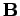which gives rise to a magnetic flux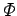linking the circuit. We expect the fluxto be directly proportional to the current, given the linear nature of the laws of magnetostatics, and the definition of magnetic flux. Thus, we can write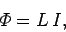(241)

where the constant of proportionality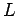is called the self inductance of the circuit. Like mutual inductance, the self inductance of a circuit is measured in units of henries, and is a purely geometric quantity, depending only on the shape of the circuit and number of turns in the circuit.

If the current flowing around the circuit changes by an amount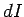in a time interval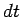then the magnetic flux linking the circuit changes by an amount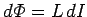in the same time interval. According to Faraday's law, an emf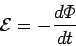(242)

is generated around the circuit. Since, this emf can also be written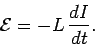(243)

Thus, the emf generated around the circuit due to its own current is directly proportional to the rate at which the current changes. Lenz's law, and common sense, demand that if the current is increasing then the emf should always act to reduce the current, and vice versa. This is easily appreciated, since if the emf acted to increase the current when the current was increasing then we would clearly get an unphysical positive feedback effect in which the current continued to increase without limit. It follows, from Eq. (243), that the self inductanceof a circuit is necessarily a positive number. This is not the case for mutual inductances, which can be either positive or negative.

Consider a solenoid of length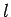and cross-sectional area. Suppose that the solenoid hasturns. When a currentflows in the solenoid, a uniform axial field of magnitude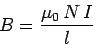(244)

is generated in the core of the solenoid. The field-strength outside the core is negligible. The magnetic flux linking a single turn of the solenoid is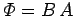. Thus, the magnetic flux linking allturns of the solenoid is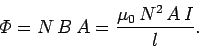(245)

According to Eq. (241), the self inductance of the solenoid is given by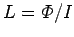, which reduces to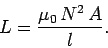(246)

Note thatis positive. Furthermore,is a geometric quantity depending only on the dimensions of the solenoid, and the number of turns in the solenoid.

Engineers like to reduce all pieces of electrical apparatus, no matter how complicated, to an equivalent circuit consisting of a network of just four different types of component. These four basic components are emfs, resistors, capacitors, and inductors. An inductor is simply a pure self inductance, and is usually represented a little solenoid in circuit diagrams. In practice, inductors generally consist of short air-cored solenoids wound from enameled copper wire.Next: Energy Stored in an Up: Inductance Previous: Mutual Inductance
Richard Fitzpatrick 2007-07-14